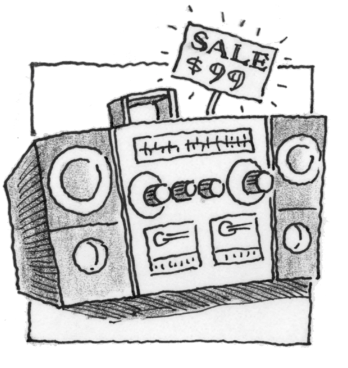### Home > APCALC > Chapter 6 > Lesson 6.1.2 > Problem6-23

6-23.

An electronics store purchases stereos from a supply company at a cost of $63$ each. They currently sell the stereos for $99$ each. At this price the store is averaging $23$ sales per week. Marketing studies show that for every $2$ decrease in price, the store will sell an additional five stereos. What price should the store use to maximize their profits?

$\text{Revenue} = \left(\text{profit}\right)\left(\text{number sold}\right)$

Calculate the current revenue:
Profit: $99$ - $63$
Number sold: $23$

Let $x =$ the number of times the price is decreased by $2$.
$R(x) = (36 − 2x)(23 + 5x)$
Optimize (that is, use derivatives to find the optimal value of $x$). But note, the question is asking about price, not $x$.